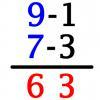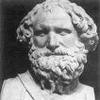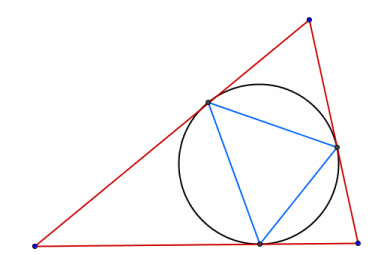#### You may also like### Vedic Sutra - All from 9 and Last from 10

Vedic Sutra is one of many ancient Indian sutras which involves a cross subtraction method. Can you give a good explanation of WHY it works?### Tournament Scheduling

Scheduling games is a little more challenging than one might desire. Here are some tournament formats that sport schedulers use.### Archimedes and Numerical Roots

The problem is how did Archimedes calculate the lengths of the sides of the polygons which needed him to be able to calculate square roots?

# Triangle Incircle Iteration

##### Age 14 to 16Challenge LevelStart with any triangle. Draw its inscribed circle (the circle which just touches each side of the triangle). Draw the triangle which has its vertices at the points of contact between your original triangle and its incircle. Now keep repeating this process starting with the new triangle to form a sequence of nested triangles and circles. What happens to the triangles?

If the angles in the first triangle are $a$, $b$ and $c$ prove that the angles in the second triangle are given (in degrees) by

$f(x) = (90 - x/2)$

where $x$ takes the values $a$, $b$ and $c$. Choose some triangles, investigate this iteration numerically and try to give reasons for what happens.

Investigate what happens if you reverse this process (triangle to circumcircle to triangle...)